Courses

# Previous year questions (2016-20) - Current Electricity (Part - 2) Notes | EduRev

## JEE : Previous year questions (2016-20) - Current Electricity (Part - 2) Notes | EduRev

The document Previous year questions (2016-20) - Current Electricity (Part - 2) Notes | EduRev is a part of the JEE Course Physics Class 12.
All you need of JEE at this link: JEE

Q 33. On interchanging the resistances, the balance point of a meter bridge shifts to the left by 10 cm. The resistance of their series combinations is 1 kΩ. How much was the resistance on the left slot before interchanging the resistances?    
(1) 990 Ω
(2) 505 Ω
(3) 550 Ω
(4) 910 Ω
Ans:
(3)
Solution: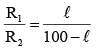(i)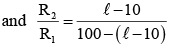(ii)
From (i) and (ii)
⇒ l = 55 cm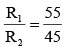(1)
and  R1 + R 2 = 1000 Ω       (iii)
from  (i) and (iii)
R = 550 Ω

Q 34. In the given circuit all resistances are of value R ohm each. The equivalent resistance between A and B is: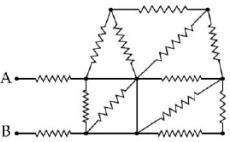(1) 5R/3
(2) 3R
(3) 5R/2
(4) 2R
Ans:
D
Solution: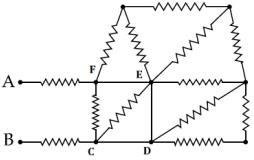Due to short circuit current will flow along
B-C-D-E-F-A
So
R eq = R + R
R eq = 2R

Q 35.  In a meter bridge, as shown in the figure, it is given that resistance Y =12.5 Ω and that the balance is obtained at a distance 39.5 cm from end A (by Jockey J). After interchanging the resistances X and Y, a new balance point is found at a distance Ifrom end A. What are the values of X and l2?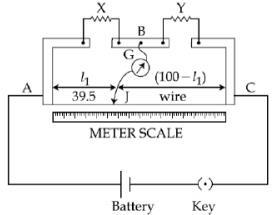(1) 19.15 Ω and 60.5 cm
(2) 19.15 Ω and 39.5 cm
(3) 8.16 Ω and 39.5 cm
(4) 8.16 Ω and 60.5 cm
Ans:
(4)
Solution:
For balanced wheat stone bridge
x(100 − l1) = y × l1
So x(100 − 39.5) = 12.5(39.5)
x = 8.16 Ω
If x and y inter changed
y(100 − l2) = xl2
12.5(100 − l2) = 8.16 l2
get l2 = 60.5 cm

Q 36. In the given circuit the current through zener diode is :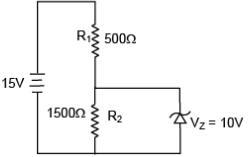(1) 3.3mA
(2) 2.5mA
(3) 5.5mA
(4) 6.7mA
Ans:
(1)
Solution: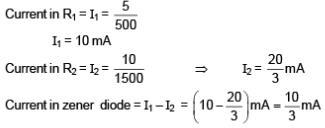Q 37.  A galvanometer with its coil resistance 25Ω requires a current of 1mA for its full deflection. In order to construct an ammeter to read up to a current of 2A the approximate value of the shunt resistance should be:    
(1) 1.25 × 10–2Ω
(2) 2.5 × 10–3Ω
(3) 2.5 × 10–2Ω
(4) 1.25 × 10–3Ω
Ans:
(1)
Solution: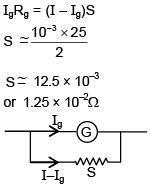Q 38. In a circuit for finding the resistance of a galvanometer by half deflection method a 6V battery and a high resistance of 11 kΩ are used. The figure of merit of the galvanometer produces a deflection of θ = 9 divisions when current flows in the circuit. The value of the shunt resistance that can cause the deflection of θ/2 is    
(1) 550Ω
(2) 220Ω
(3) 55Ω
(4) 110Ω
Ans:
(4)
Solution: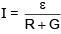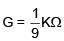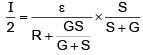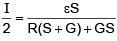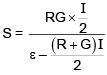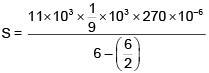S = 110Ω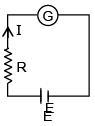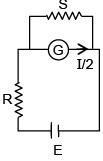Q 39. In the following circuit the switch S is closed at t = 0. The charge on the capacitor C1 as a function of time will be given by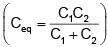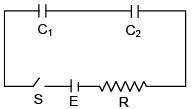(1) C1E[1 – exp(–tR/C1)]
(2) CeqE exp(–t/RCeq)
(3) CeqE [1 – exp(–t/RCeq)]
(4) C2E[1 – exp(–t/RC2)]
Ans:
C
Solution:
q = CeqE [1 – e–t/RCeq)]
Both capacitor will have same charge as they are connected in series.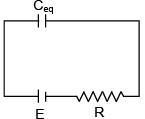Q 40. Which of the following statements is false?
(1) A rheostat can be used as a potential divider
(2) Kirchhoff’s second law represents energy conservation
(3) Wheatstone bridge is the most sensitive when all the four resistances are of the same order of magnitude
(4) In a balanced Wheatstone bridge if the cell and the galvanometer are exchanged, the null point is disturbed    
Ans:
(4)
Solution:
In a balanced Wheatstone bridge, the null point remains unchanged even if cell and galvanometer are interchanged.

Q 41. When a current of 5 mA is passed through a galvanometer having a coil of resistance 15 Ω, it shows full scale deflection. The value of the resistance to be put in series with the galvanometer to convert it into a voltmeter of range 0-10 V is    
(1) 2.535 × 103
(2) 4.005 × 103
(3) 1.985 × 103
(4) 2.045 × 103
Ans:
(3)
Solution:
ig = 5 × 10–3 A
G = 15 Ω
Let series resistance be R.
V = ig (R + G)
10 = 5 × 10–3 (R + 15)
R = 2000 – 15 = 1985 = 1.985 × 103

Q 42.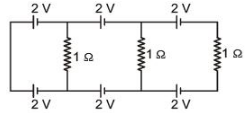In the above circuit the current in each resistance is    
(1) 0.5 A
(2) 0 A
(3) 1 A
(4) 0.25 A
Ans:
(2)
Solution:
The potential difference in each loop is zero.
∴ No current will flow.

Q 43. A potentiometer PQ is set up to compare two resistances as shown in the figure. The ammeter A in the circuit reads 1.0 A when two way key K3 is open. The balance point is at a length l1 cm from P when two way key K3 is plugged in between 2 and 1, while the balance point is at a length l2 cm from P when key K3 is plugged in between 3 and 1. The ratio of the two resistance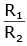2 is found to be -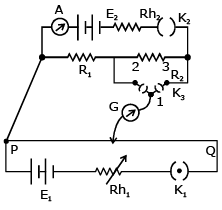(1)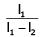(2)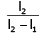(3)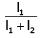(4)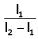Ans:
(4)
Solution:
When key is at point
V1 = iR1 = xl1
when key is at (3)
V2 = i (R1 + R2) = xl2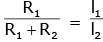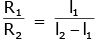Q 44. A 9 V battery with internal resistance of 0.5 W is connected across an infinite network as shown in the figure. All ammeters A1, A2, A3 and voltmeter V are ideal.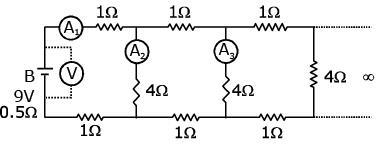Choose correct statement.    
(1) Reading of A1 is 18 A
(2) Reading of V is 9 V
(3) Reading of V is 7 V
(4) Reading of A1 is 2 A
Ans:
(4)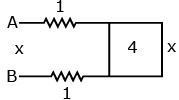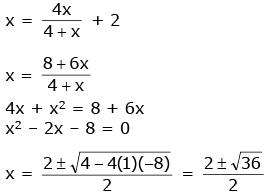Q 45. In a meter bridge experiment resistances are connected as shown in the figure. Initially resistance P = 4 Ω and the neutral point N is at 60 cm from A. Now an unknown resistance R is connected in series to P and the new position of the neutral point is at 80 cm from A. The value of unknown resistance R is -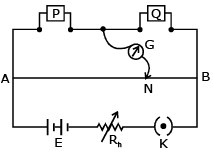(1)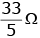(2)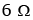(3)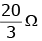(4) 7 Ω
Ans: (3)
Initially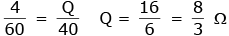Finally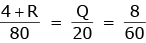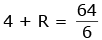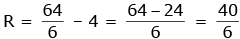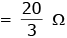Q 46. A uniform wire of length I and radius r has a resistance of 100 Ω. It is recast into a wire of radius r/2. The resistance of new wire will be -     
(1) 1600 Ω
(2) 100 Ω
(3) 200 Ω
(4) 400 Ω
Ans: (1)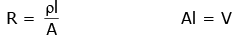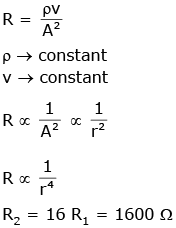Q 47. The figure shows three circuits I, II and III which are connected to a 3V battery. If the powers dissipated by the configurations I, II and III are P1, P2 and P3 respectively, then -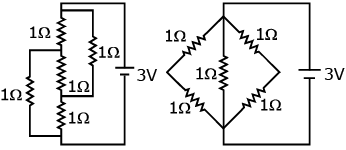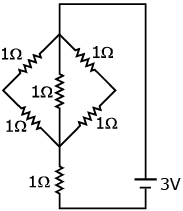(2017)
(1) P2 > P1 > P3
(2) P1 > P2 > P3
(3) P3 > P2 > P1
(4) P1 > P3 > P2
Ans: (1)
Solution: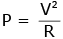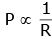R1 = 1
R2 = 1/2
R3 = 2
P2 > P1 > R3

Q 48. A combination of capacitors is set up as shown in the figure. The magnitude of the electric field due to a point charge Q (having a charge equal to the sum of the charges on the 4 μF and 9 μF capacitors), at a point distant 30 m from it, would equal: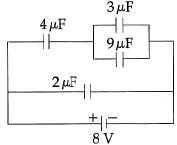(1) 240 N/C
(2) 360 N/C
(3) 420 N/C
(4) 480 N/C
Ans:
(3)
Solution:Charges on 3 μF = 3 μF x δV = 24 μC∴ Charge on 4 μF = Charge on 12 μF = 24 μC
Charge of 3 μF = 3 μF ´ 2V = 6 μC
Charge of 9 μF = 9 μF ´ 2V = 18 mC
Charge on 4 μF + Charge on 9 μF = (24 + 18) μF = 42 μC
∴ Electric field at 30 m = 9 x 103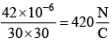Q 49. A galvanometer having a coil resistance of 100 Ω gives a full scale deflection, when a current of 1 mA is passed through it. The value of the resistance, which can convert this galvanometer into ammeter giving a full scale deflection for a current of 10 A is :    
(1) 0.01 Ω
(2) 2 Ω
(3) 0.1 Ω
(4) 1 Ω
Ans:
(1)
Solution:
Maximum voltage that can be applied across the galvanometer coil = 100 Ω x 10-3 A = 0.1 V.
If RS is the shunt resistance :
RS x 10 A = 0.1 V
⇒ RS = 0.01 Ω

Q 50.  Three capacitors each of 4μF are to be connected in such a way that the effective capacitance is 6μF.
This can be done by connecting them :
(1) all in series
(2) two in parallel and one in series
(3) two in series and one in parallel
(4) all in parallel
Ans:
(3)
Solution: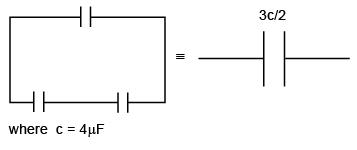Q 51. In the circuit shown, the resistance r is a variable resistance. If for r =fR, the heat generation in r is maximum then the value of f is :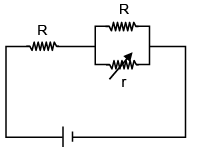(2016)
(1) 1
(2) 3/4
(3) 1/4
(4) 1/2
Ans:
(4)
Solution: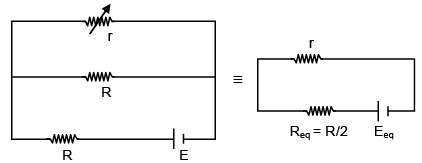to get maximum heat generation from r.
r = Req = R/2

Q 52. To know the resistance G of a galvanometer by half deflection method, a battery of emf VE and resistance R is used to deflect the galvanometer by angle q. If a shunt of resistance S is needed to gat half deflection then G, R and S are related by the equation:     (2016)
(1) 2S = G
(2) 2G = S
(3) S(R+G) = RG
(4) 2S(R+G) = RG
Ans: (3)
Solution: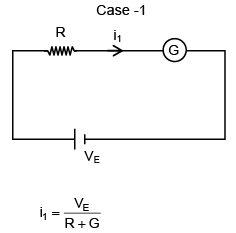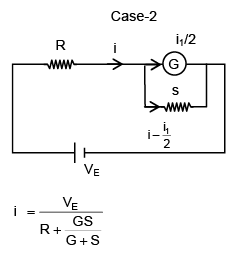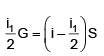i1 (G+S) = 2 i S
substituting i1 and i we get
S(R+G) = RG

Q 53. A 50 Ω resistance is connected to a battery of 5 V. A galvanometer of resistance 100 Ω is to be used as ammeter to measure current through the resistance. For this, a resistance rs is connected to the galvanometer. Which of the following connections should be employed if the measured current is within 1% of the current without the ammeter in the circuit ?    
(1) rs = 0.5 Ω in parallel with the galvanometer
(2) rs = 0.5 Ω in series with the galvanometer
(3) rs = 1 Ω in series with galvanometer
(4) rs = 1 Ω in parallel with galvanometer
Ans:
(1)
Solution:
Its clear, shunt should be applied in parallel and least the shunt resistance, better the ammeter is.

Q 54. Figure shows a network of capacitors where the number indicates capacitances in micro Farad. The value of capacitance C if the equivalent capacitance between point A and B is to be 1mF is: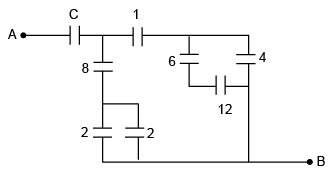(2016)
(1) 33/23 μF
(2) 31/23 μF
(3) 32/23 μF
(4) 34/23 μF
Ans:  (3)
Solution: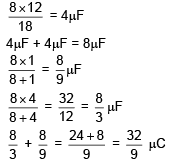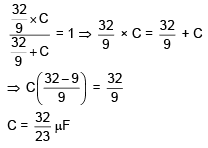Q 55. A galvanometer has a 50 division scale. Battery has no internal resistance. It is found that there is deflection of 40 divisions when R = 2400 Ω. Deflection becomes 20 divisions when resistance taken form resistance box is 4900 Ω. Then we can conclude: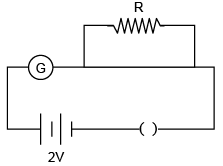(1) Resistance required on R.B. For a deflection of 10 divisions is 9800 W.
(2) Full scale deflection current is 2 μA.
(3) Current sensitivity of galvanometer is 20μA/ division .
(4) Resistance of galvanometer is 200 Ω.
Ans: Bonus

Q 56. The resistance of an electrical toaster has a temperature dependence given by R (T) = R0 [1+α(T- T0] in its range of operation. At T0 = 300 K, R = 100 Ω. And at T = 500 K, R = 120 Ω. The toaster is connected to a voltage source at 200 V and its temperature is raised at a constant rate form 300 to 500 K in 30s. The total work done in raising the temperature is:    (2016)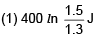(2) 3000 J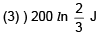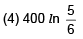Ans: Bonus
Solution: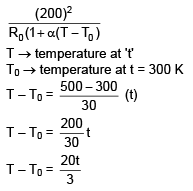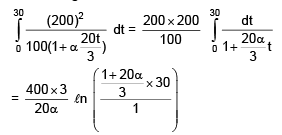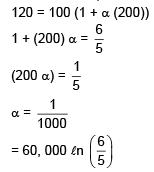Offer running on EduRev: Apply code STAYHOME200 to get INR 200 off on our premium plan EduRev Infinity!

,

,

,

,

,

,

,

,

,

,

,

,

,

,

,

,

,

,

,

,

,

;